# Algebra II : Exponents

## Example Questions

← Previous 1 3 4 5 6 7 8 9 76 77

### Example Question #1 : Negative Exponents

Simplify the following expression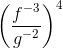Possible Answers: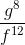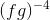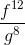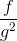Correct answer:Explanation: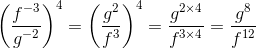### Example Question #2 : Negative Exponents

Simplify the following expression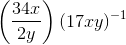Possible Answers: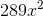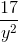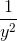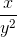Correct answer:Explanation: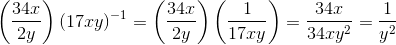### Example Question #3 : Negative Exponents

Simplify the following expression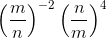Possible Answers: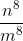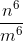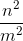Correct answer:Explanation: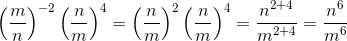### Example Question #4 : Negative Exponents

Simplify the following expression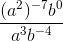Possible Answers: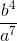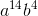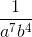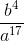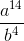Correct answer:Explanation: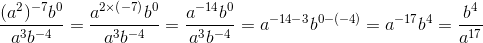### Example Question #5 : Negative Exponents

Solve for: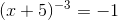Possible Answers: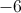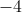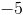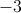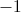Correct answer:Explanation:

Raise both sides of the equation to the inverse power ofto cancel the exponent on the left hand side of the equation.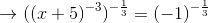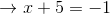Subtractfrom both sides: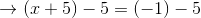### Example Question #6 : Negative Exponents

Represent the fraction using only positive exponents: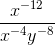Possible Answers: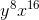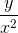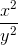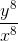Correct answer:Explanation:Negative exponents are the reciprocal of their positive counterpart. For example: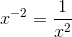Therefore: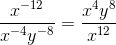This simplifies to:### Example Question #7 : Negative Exponents

Solve the equation for n: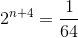Possible Answers: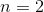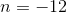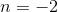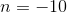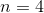Correct answer:Explanation:

Rewrite the right-hand-side so that each side has the same base: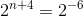Use the Property of Equality for Exponential Functions: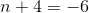Solving for:### Example Question #8 : Negative Exponents

What is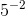the same as?

Possible Answers: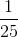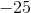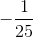Correct answer:Explanation:

While a positive exponent says how many times to multiply by a number, a negative exponent says how many times to divide by the number.

To solve for negative exponents, just calculate the reciprocal.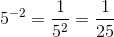### Example Question #9 : Negative Exponents

Solve: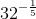Possible Answers: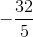Correct answer:Explanation:

To evaluate a negative exponent, convert the exponent to positive by taking the inverse.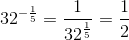### Example Question #10 : Negative Exponents

Simplify: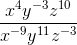Possible Answers: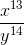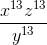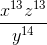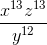Correct answer:Explanation:

To simplify this expression, first make all of the negative exponents positive. That means putting them in the opposite position (if they're in the numerator, put it in the denominator and vice versa).

It should then look like: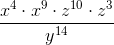.

Then, combine like terms. Remember, if bases are the same, add exponents!

Therefore, your answer is:← Previous 1 3 4 5 6 7 8 9 76 77

### All Algebra II Resources